Courses

# NCERT Solutions(Part - 1) - Integers Class 7 Notes | EduRev

## Class 7: NCERT Solutions(Part - 1) - Integers Class 7 Notes | EduRev

The document NCERT Solutions(Part - 1) - Integers Class 7 Notes | EduRev is a part of the Class 7 Course Mathematics (Maths) Class 7.
All you need of Class 7 at this link: Class 7

Ques 1: Following number line shows the temperature in degree celsius (°C) at different places on a particular day.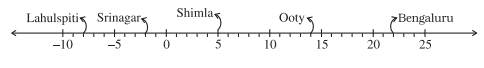(a) Observe this number line and write the temperature of the places marked on it.
(b) What is the temperature difference between the hottest and the coldest places among the above?
(c) What is the temperature difference between Lahaul Spiti and Srinagar?
(d) Can we say temperature of Srinagar and Shimla taken together is less than the temperature at Shimla? Is it also less than the temperature at Srinagar
Ans:
(a) The temperature of the places marked on it is:

 Places Temperature Places Temperature Bangalore 22°C Srinagar -2°C Ooty 14°C Lahaul Spiti -8°C Shimla 5°C

(b) The temperature of the hottest place Bangalore = 22°C
The temperature of the coldest place Lahaul Spiti = -8°C
Difference = 22°C - (-8°C) = 22°C + 8°C = 30°C
(c) The temperature of Srinagar = -2°C
The temperature of Lahaul Spiti = -8°C
Difference = -2°C + (-8°C) = -2°C -8°C = -6°C
(d) The temperature of Srinagar and Shimla = 5°C + (-2°C) = 5°C -2°C = 3°C
The temperature at Shimla = 5°C
Therefore, 3°C < 5°C
Thus, temperature of Srinagar and Shimla taken together is less than the temperature at Shimla.
Now, Temperature of Srinagar = -2°C
∴ 3°C > -2°C
No, It is not less than the temperature at Srinagar.

Ques 2: In a quiz, positive marks are given for correct answers and negative marks are given for incorrect answers. If jack’s scores in five successive rounds were 25, -5, -10, 15 and 10, what was his total at the end?
Ans: Jack's scores in five successive rounds are 25, -5, -10, 15 and 10.
Total marks got by Jack = 25 + (-5) + (-10) + 15 + 10
= 25 - 15 + 25 = 35
∴ Jack’s total score at the end is 35.

Ques 3: At Srinagar temperature was -5°C on Monday and then it dropped by 2°C on Tuesday. What was the temperature of Srinagar on Tuesday? On Wednesday, it rose by 4°C. What was the temperature on this day?
Ans: On Monday, temperature at Srinagar = -5°C
On Tuesday, temperature dropped by = 2°C
∴ Temperature on Tuesday = -5°C - 2°C = -7°C
On Wednesday, temperature rose up by = 4°C
∴  Temperature on Wednesday = -7°C + 4°C = -3°C
Thus, temperature on Tuesday and Wednesday was -7°C and -3°C respectively.

Ques 4: A plane is flying at the height of 5000 m above the sea level. At a particular point, it is exactly above a submarine floating 1200 m below the sea level. What is the vertical distance between them?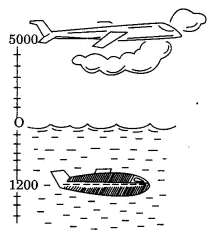Ans: Height of a plane above the sea level = 5000 m
Floating a submarine below the sea level = -1200 m
∴ The vertical distance between the plane and the submarine
= 5000 - (- 1200) = 6200 m
Thus, the vertical distance between the plane and the submarine is 6200 m.

Ques 5: Mohan deposits ₹ 2,000 in his bank account and withdraws ₹ 1,642 from it, the next day. If withdrawal of amount from the account is represented by a negative integer, then how will you represent the amount deposited? Find the balance in Mohan’s account after the withdrawal.
Ans: Withdrawal of amount from the account is represented by a negative integer.
Then, deposit of amount to the account is represented by a positive integer.
From the question,
Total amount deposited in bank account by the Mohan = ₹ 2000
Total amount withdrawn from the bank account by the Mohan = – ₹ 1642
Balance in Mohan’s account after the withdrawal = amount deposited + amount withdrawn
= ₹ 2000 + (-₹ 1642)
= ₹ 2000 – ₹ 1642
= ₹ 358
Hence, the balance in Mohan’s account after the withdrawal is ₹ 358

Ques 6: Rita goes 20 km towards east from a point A to the point B. From B, she moves 30 km towards west along the same road. If the distance towards east is represented by a positive integer then, how will you represent the distance travelled towards west? By which integer will you represent her final position from A?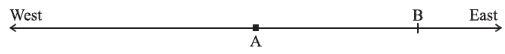Ans: According to the number line, Rita moves towards east is represented by a positive integer. But she moves in opposite direction means Rita moves west, is represented by negative integer.
Distance from A to B = 20 km
Distance from B to C = 30 km
Distance from A to C = 20 – 30 = –10 km
Thus, Rita is at final position from A to C is –10 km.

Ques 7: In a magic square each row, column and diagonal have the same sum. Check which of the following is a magic square.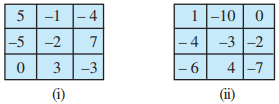Ans: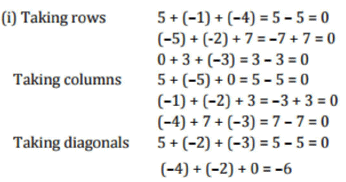This box is not a magic square because all the sums are not equal.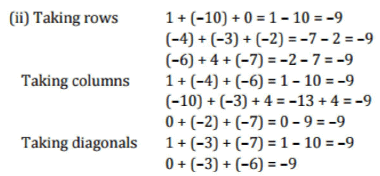This box is magic square because all the sums are equal.

Ques 8: Verify a -(−b) = a + b for the following values of a and b.
(i) a = 21, b = 18
(ii) a = 118, b = 125
(iii) a = 75, b = 84
(iv) a = 28, b = 11
Ans: (i) Given: a = 21,b = 18
To Verify, a -(-b) = a + b
Putting the values in L.H.S. = a - (- b) = 21 - (- 18) = 21 + 18 = 39
Putting the values in R.H.S. = a + b = 21 + 18 = 39
Since, L.H.S. = R.H.S
Hence, verified.
(ii) Given: a - 118,6 = 125
To Verify, a - (- b) = a + b
Putting the values in L.H.S. = a - (- b) = 118 -(-125) = 118 + 125 = 243
Putting the values in R.H.S. = a + b = 118 + 125 = 243
Since, L.H.S. = R.H.S
Hence, verified.

(iii) Given: a = 75, b = 84
To Verify, a - (-b) = a + b
Putting the values in L.H.S. = a - (- b) = 7 5 - (- 84) = 75 + 84 = 159
Putting the values in R.H.S. = a + b = 75 + 84 = 159
Since, L.H.S. = R.H.S
Hence, verified.
(iv) Given: a = 28, b = 11
To Verify, a - (- b) - a + b
Putting the values in L.H.S. = a -(- b) = 28 - (- 11) = 28 + 11 = 39
Putting the values in R.H.S. = a + b = 28 + 11 = 39
Since, L.H.S. = R.H.S
Hence, verified.

Ques 9: Use the sign of >, < or = in the box to make the statements true.
Ans: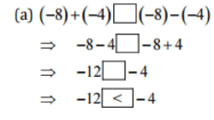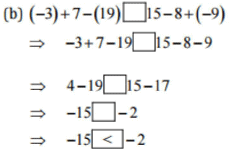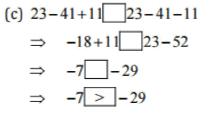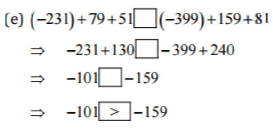Ques 10: A water tank has steps inside it. A monkey is sitting on the topmost step (i.e., the first step). The water level is at the ninth step.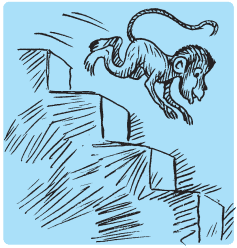(i) He jumps 3 steps down and then jumps back 2 steps up. In how many jumps will he reach the water level?
(ii) After drinking water, he wants to go back. For this, he jumps 4 steps up and then jumps back 2 steps down in every move. In how many jumps will he reach back the top step?
(iii) If the number of steps moved down is represented by negative integers and the number of steps moved up by positive integers, represent his moves in part (i) and (ii) by completing the following;
(a) -3 + 2 -… = -8
(b) 4 -2 + … = 8
In (a) the sum (-8) represents going down by eight steps. So, what will the sum 8 in (b) represent?
Ans:
(i) He jumps 3 steps down and jumps back 2 steps up.
Following number ray shows the jumps of monkey: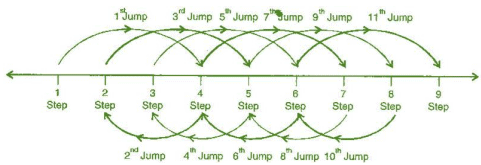First jump = 1 + 3 = 4 steps
Second jump = 4 - 2 = 2 steps
Third jump = 2 + 3 = 5 steps
Fourth jump = 5 - 2 = 3 steps
Fifth jump = 3 + 3 = 6 steps
Sixth jump = 6 - 2 = 4 steps
Seventh jump = 4 + 3 = 7 steps
Eighth jump = 7 - 2 = 5 steps
Ninth jump = 5 + 3 = 8 steps
Tenth jump = 8 - 2 = 6 steps
Eleventh jump = 6 + 3 = 9 steps
He will reach ninth steps in 11 jumps.
(ii) He jumps four steps and then jumps down 2 steps. Following number ray shows the jumps of monkey: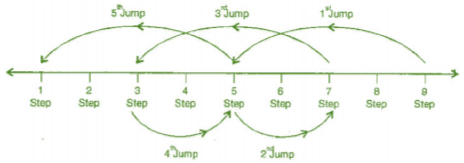Let us consider steps moved down are represented by positive integers and then, steps moved up are represented by negative integers.
Initially monkey is sitting on the ninth step i.e., at the water level
In 1st jump monkey will be at step = 9 + (-4) = 9 – 4 = 5 steps
In 2nd jump monkey will be at step = 5 + 2 = 7 steps
In 3rd jump monkey will be at step = 7 + (-4) = 7 – 4 = 3 steps
In 4th jump monkey will be at step = 3 + 2 = 5 steps
In 5th jump monkey will be at step = 5 + (-4) = 5 – 4 = 1 step
∴Monkey took 5 jumps to reach back the top step i.e., first step.
(iii)
From the question, it is given that
If the number of steps moved down is represented by negative integers and the number of steps moved up by positive integers.
Monkey moves in part (i)
= - 3 + 2 - ……….. = - 8
Then LHS = - 3 + 2 - 3 + 2 - 3 + 2 - 3 + 2 - 3 + 2 - 3
= - 18 + 10
= - 8
RHS = -8
∴ Moves in part (i) represents monkey is going down 8 steps. Because negative integer.
Now,
Monkey moves in part (ii)
= 4 - 2 + ……….. = 8
Then LHS = 4 - 2 + 4 - 2 + 4
= 12 - 4
= 8
RHS = 8
∴Moves in part (ii) represents monkey is going up 8 steps. Because positive integer.

The document NCERT Solutions(Part - 1) - Integers Class 7 Notes | EduRev is a part of the Class 7 Course Mathematics (Maths) Class 7.
All you need of Class 7 at this link: Class 7Use Code STAYHOME200 and get INR 200 additional OFF Use Coupon Code
All Tests, Videos & Notes of Class 7: Class 7

### Top Courses for Class 7## Mathematics (Maths) Class 7

161 videos|237 docs|45 tests

### Top Courses for Class 7Track your progress, build streaks, highlight & save important lessons and more!

,

,

,

,

,

,

,

,

,

,

,

,

,

,

,

,

,

,

,

,

,

;